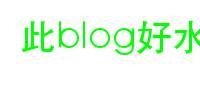### 为什么要Freetype？

FreeType是一个字形图`glyph image`产生工具，整个库的主要功能点集中在以下两点：

• 解析几乎是所有的字体格式，例如常见的`ttf，otf`
• 根据输入的单个Unicode返回该字的`glyph`也就是字形图。

• 颜色。
• 处理字符串。

### 我要写什么

• 输出字图
• 渲染一个句子：调整字色，字号，字间距（tracking）

### 编译

Freetype的编译方法(Windows党退散吧)相当简单，仅仅三步：

``````./configure
make
make install
``````

``````./configure && make
ar rcs output.a objs/*.o
``````

### 输出字图 (`glyph`)

#### 初始化Freetype

``````extern "C"
{
#include <ft2build.h>
#include FT_FREETYPE_H
}
/* 省略X行 */
FT_Library library; // 声明了Lib
FT_Init_FreeType(&library); // 初始化 返回0为成功
``````

#### 根据字体初始化Face

``````FT_Face face;
// 在Mac OS上字体放在 /Library/Fonts
error = FT_New_Face( library,
"/Library/Fonts/华文黑体.ttf",
0,
&face);
FT_Set_Pixel_Sizes(face, 0, 16); // 设立字体为16px
``````

#### 获得字图

``````wchar c = "哟";
/*
* 这一步中实际上发生了几件事。
* FT先根据输入，获得Unicode然后获得对应的glyph id。
* 接着用glyph id渲染出了一张bitmap
*/
FT_GlyphSlot slot = face->glyph; // 输出结果在 slot->bitmap
``````

#### 输出

``````auto bitmap = &slot->bitmap;
int rows = bitmap->rows; // 获得字高
int cols = bitmap->width; // 获得字宽
slot->bitmap[i * cols + j]; // 获得第i行第j列像素值
``````

``````/*
* 这里使用了OpenCV的cv::Mat作为输出
*/
Mat ret(100, 100, CV_8UC4, {255, 255, 255, 0});
/*
* 选定画的起点
*/
int pen_x = 50;
int pen_y = 50;
auto& bitmap = &slot->bitmap;
int rows = bitmap->rows; // 字宽
int cols = bitmap->width; // 字高
for (int i = 0; i < rows; i++) {
for (int j = 0; j < cols; j++) {
int ty = pen_y + i;
int tx = pen_x + j;
if (ty >= ret.cols || tx >= ret.rows ||
ty < 0 || tx < 0)
continue;
/*
* 填充
*/
ret.at<Vec4b>(ty, tx) =
bitmap->buffer[i * cols + j];
}
}
/* 输出成图片 */
imwrite("somepic.jpg", ret);
``````

###渲染句子

#### 字色

``````// 这样渲染出的字就是纯绿, 其他的部分都是透明的
Mat ret(100, 100, CV_8UC4, {0, 255, 0, 0});
``````

#### 字间距

``````int advance = (slot->advance.x >> 6);
``````

``````(slot->advance.x >> 6) - slot->bitmap.width;
``````

#### Demo代码

``````// 略去之前的初始化代码
int error = FT_Set_Pixel_Sizes(face, 0, 36);
if (error) {
log(logFATAL) << "yo";
}
Mat ret(100, 200, CV_8UC4, {0, 255, 0, 0});
int x = 20, y = 50; // Position of base line
const wstring s = L"此blog好水";
int len = s.length();
auto draw = [&ret] (auto* bitmap, int sx, int sy) {
int rows = bitmap->rows;
int cols = bitmap->width;
for (int i = 0; i < rows; i++) {
for (int j = 0; j < cols; j++) {
int ty = sy + i;
int tx = sx + j;
if (ty >= ret.rows || tx >= ret.cols ||
ty < 0 || tx < 0)
continue;
ret.at<Vec4b>(ty, tx) =
bitmap->buffer[i * cols + j];
}
}
};
for (int i = 0; i < len; i++) {
####结果